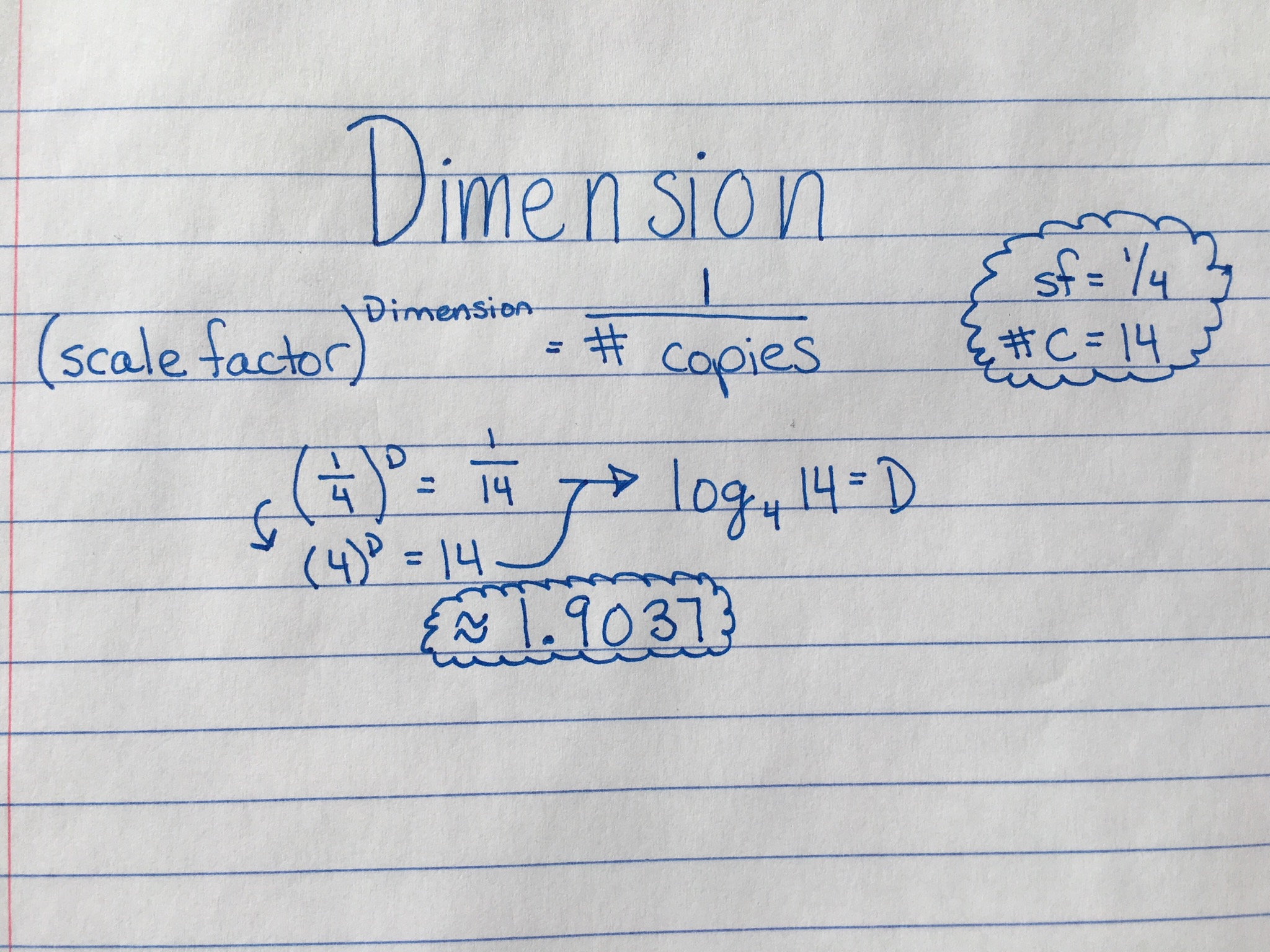# Snowflake Carpet Fractal

## Project Idea

I am calling this the snowflake carpet fractal because the higher the levels go for this fractal, the more it looks like a snowflake.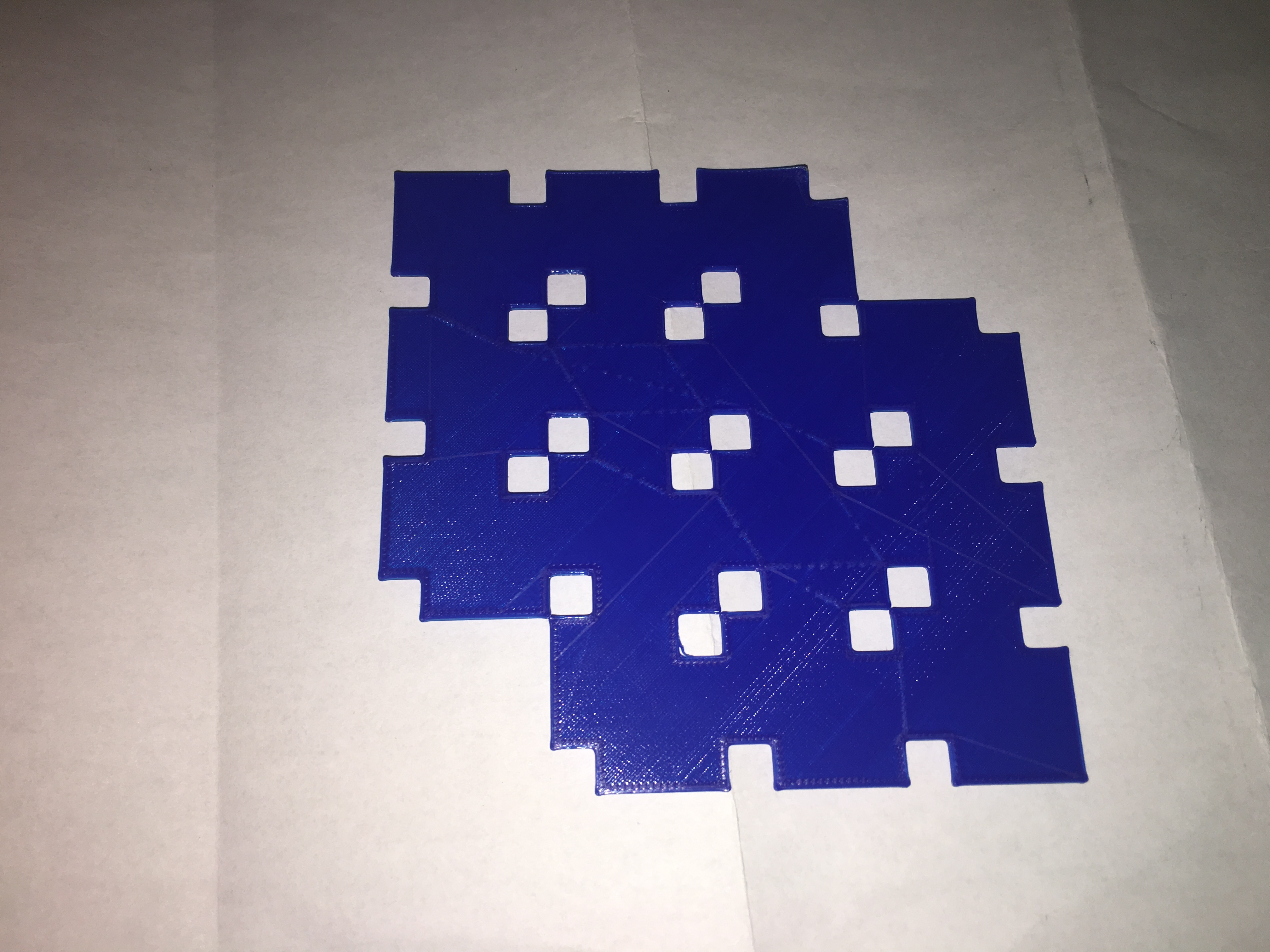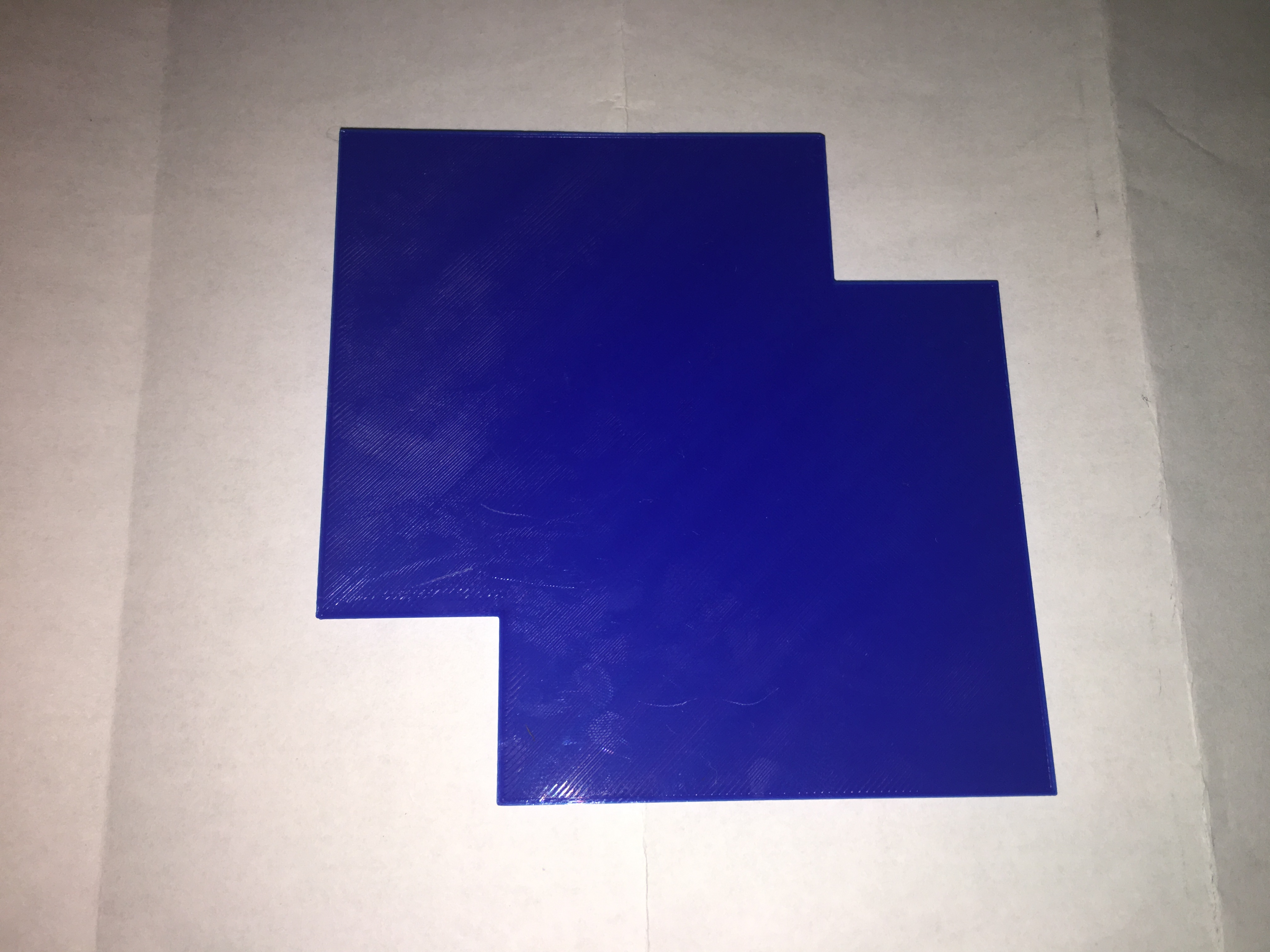To make my carpet I took out (0,0) and (3,3).

## Surface Area

Below is the equation I used to calculate the surface area of level 1, 2, and 3.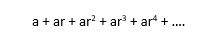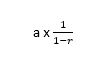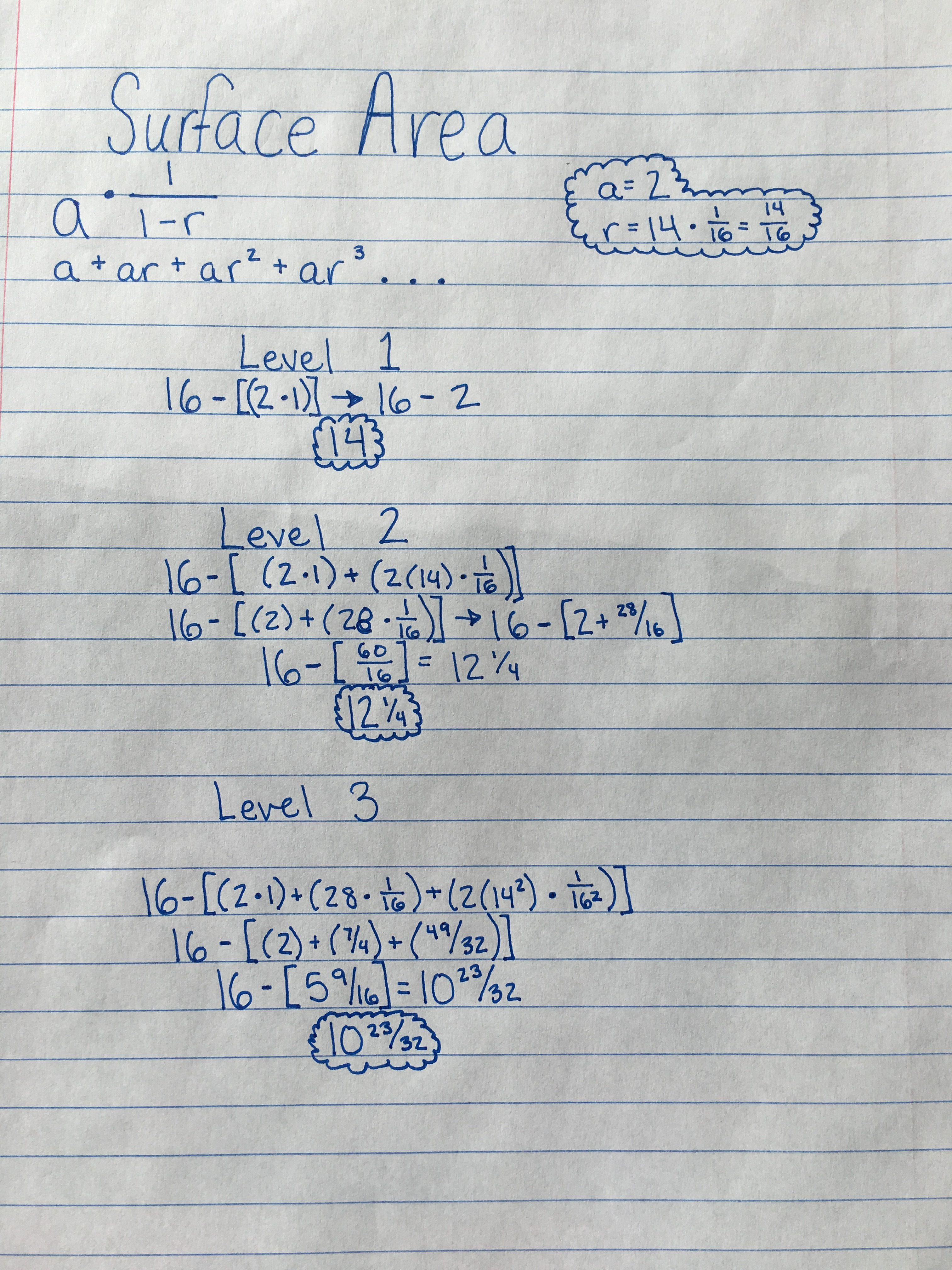## Dimension

This is the equation I used to solve for dimension.  The scaling factor was 1/4 and the number of copies was 14.